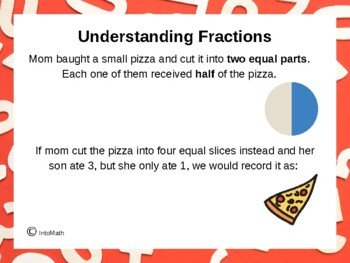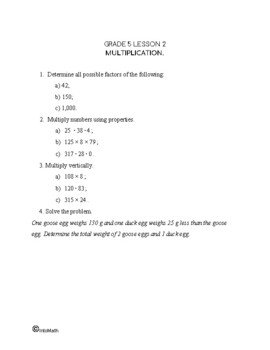CA$2.00 In this Grade 5 lesson you will learn about simple fractions and their properties. A fraction is a part of a whole. It has a numerator and a denominator. The numerator above the fraction line (bar) is used to count the parts out of the total. The number below the fraction line (bar) is called the denominator. It is used to name the total number of parts (fifth, tenth, thousandth, etc). The denominator can never be 0, because we cannot divide by 0. We can compare fractions, order them and perform various operations. You will learn and practice how to add and subtract fractions with the same denominators, as well as how to compare them. For example, you will discover that when the denominators are the same, the largest fraction is the one with the most parts (only comparing the numerators).Grade 5 Workbook (16 pages with answers) CA$4.00

The lesson teaches how to identify equivalent fractions and how to reduce them to lowest terms. For example, 2 pieces our of 8 is the same as 1 piece out of 4. Thus, if we record these relationships as fractions they would be equivalent. Equivalent means “equal”. One can be reduced to become the other.

Fractions are frequently used in everyday life and many more complex mathematical concepts are based on this concept. For example, splitting or measuring something.

Fractions go hand in hand with decimals and percent and you will discover their relationships in the upcoming lessons.

### Fractions short animation

#### Grade 5 Simple Fractions Quiz